# General Chemistry M R NaimiJamal Faculty of Chemistry

• Slides: 76General Chemistry M. R. Naimi-Jamal Faculty of Chemistry Iran University of Science & TechnologyContents 13 -1 13 -2 13 -3 13 -4 13 -5 13 -6 13 -7 The Nature of Aqueous Solutions Precipitation Reactions Acid-Base Reactions Oxidation-Reduction: Some General Principles Balancing Oxidation-Reduction Equations Oxidizing and Reducing Agents Stoichiometry of Reactions in Aqueous Solutions: Titrations13 -1 The Nature of Aqueous Solutions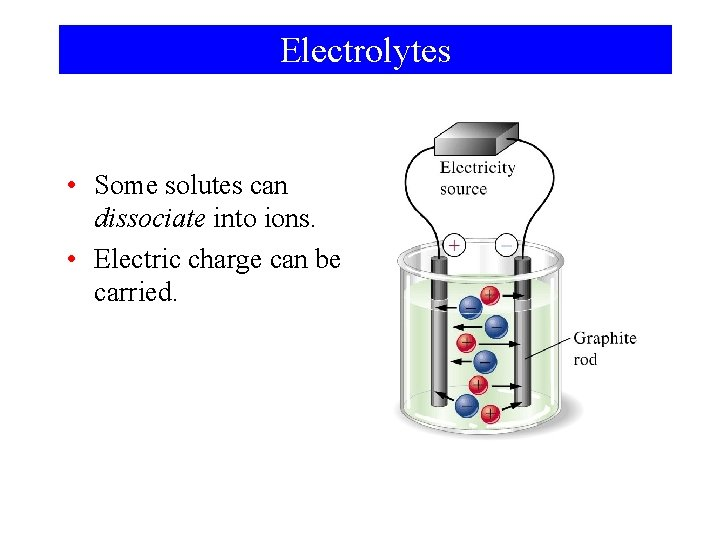Electrolytes • Some solutes can dissociate into ions. • Electric charge can be carried.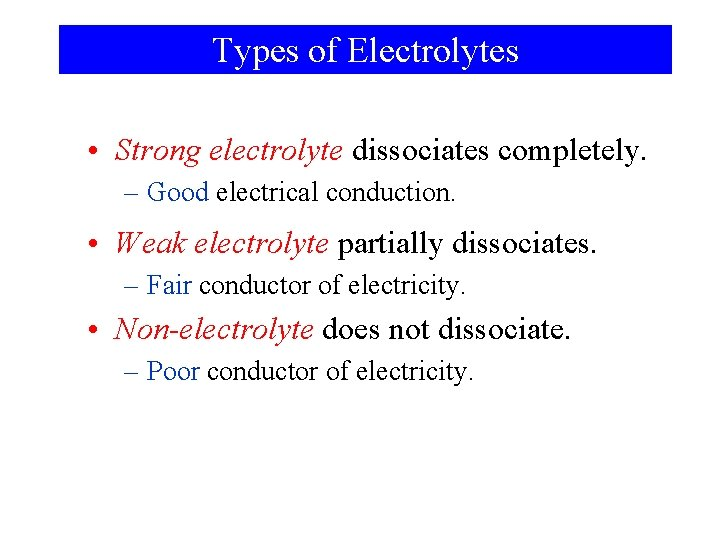Types of Electrolytes • Strong electrolyte dissociates completely. – Good electrical conduction. • Weak electrolyte partially dissociates. – Fair conductor of electricity. • Non-electrolyte does not dissociate. – Poor conductor of electricity.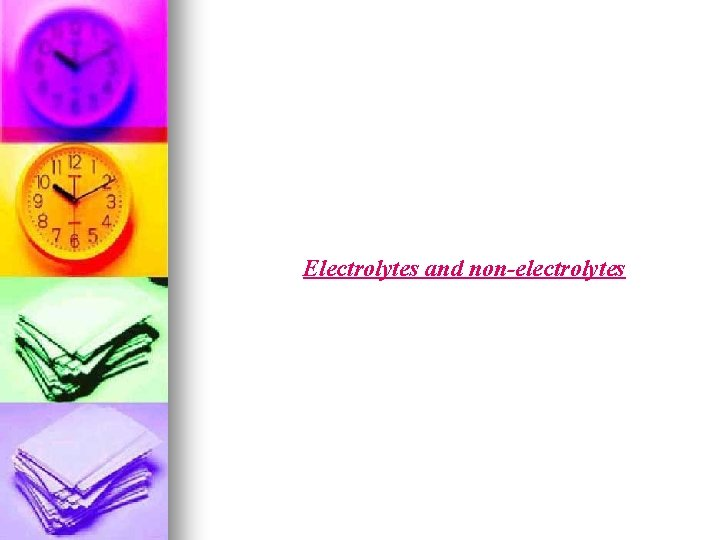Electrolytes and non-electrolytes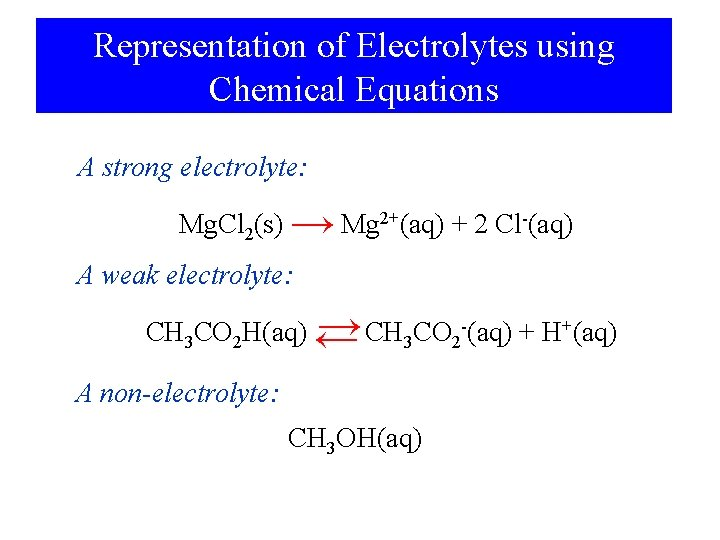Representation of Electrolytes using Chemical Equations A strong electrolyte: Mg. Cl 2(s) → Mg 2+(aq) + 2 Cl-(aq) A weak electrolyte: → CH 3 CO 2 -(aq) + H+(aq) CH 3 CO 2 H(aq) ← A non-electrolyte: CH 3 OH(aq)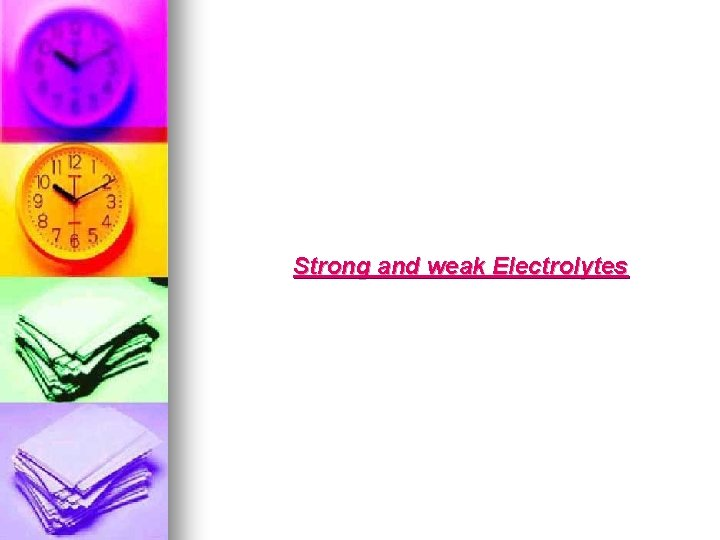Strong and weak ElectrolytesNotation for Concentration Mg. Cl 2(s) → Mg 2+(aq) + 2 Cl-(aq) In 0. 0050 M Mg. Cl 2: Stoichiometry is important. In solution: [Mg 2+] = 0. 0050 M [Cl-] = 0. 0100 M [Mg. Cl 2] = 0 M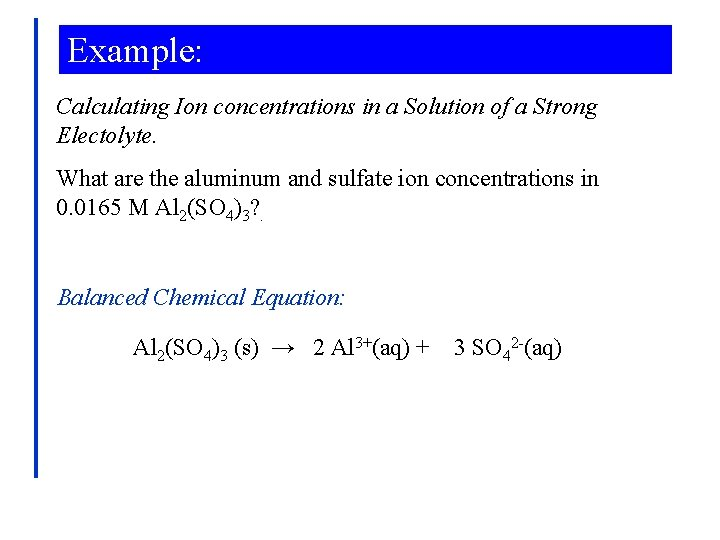Example: Calculating Ion concentrations in a Solution of a Strong Electolyte. What are the aluminum and sulfate ion concentrations in 0. 0165 M Al 2(SO 4)3? . Balanced Chemical Equation: Al 2(SO 4)3 (s) → 2 Al 3+(aq) + 3 SO 42 -(aq)Example: Aluminum Concentration: 0. 0165 mol Al 2(SO 4)3 2 mol Al 3+ [Al] = × 1 L 1 mol Al 2(SO 4)3 = 0. 0330 M Al 3+ Sulfate Concentration: [SO 4 2 -] 0. 0165 mol Al 2(SO 4)3 3 mol SO 42= × = 0. 0495 M SO 421 L 1 mol Al 2(SO 4)3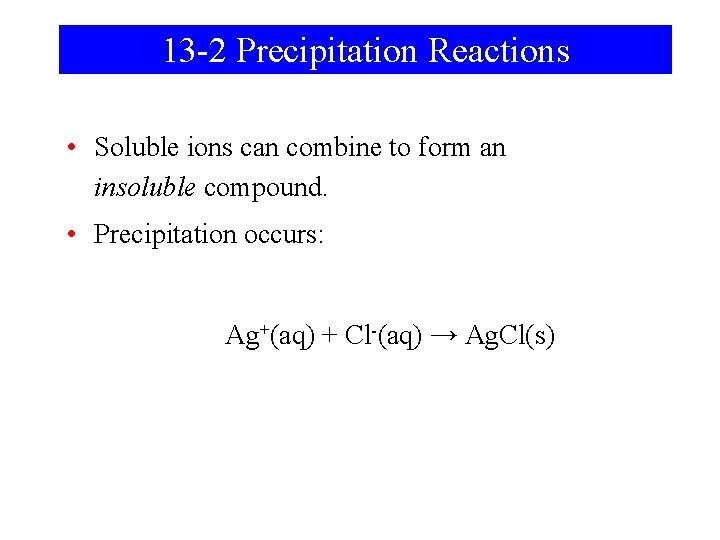13 -2 Precipitation Reactions • Soluble ions can combine to form an insoluble compound. • Precipitation occurs: Ag+(aq) + Cl-(aq) → Ag. Cl(s)Net Ionic Equation Overall Precipitation Reaction: Ag. NO 3(aq) +Na. I (aq) → Ag. I(s) + Na. NO 3(aq) Complete ionic equation: Spectator ions Ag+(aq) + NO 3 -(aq) + Na+(aq) + I-(aq) → Ag. I(s) + Na+(aq) + NO 3 -(aq) Net ionic equation: Ag+(aq) + I-(aq) → Ag. I(s)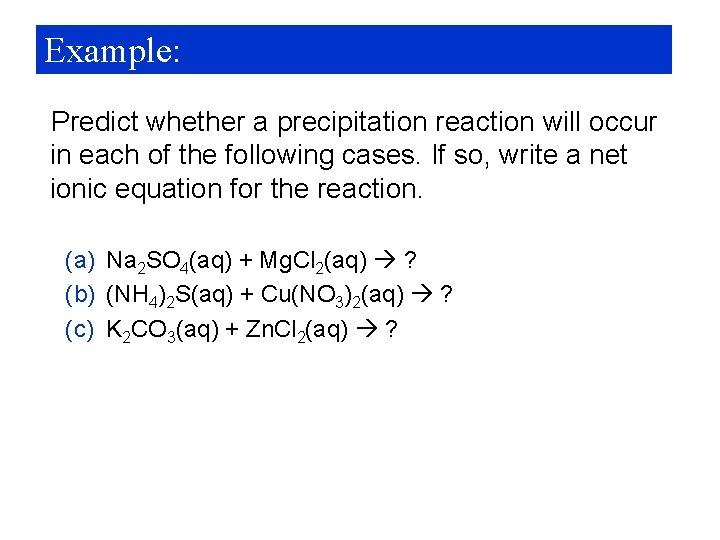Example: Predict whether a precipitation reaction will occur in each of the following cases. If so, write a net ionic equation for the reaction. (a) Na 2 SO 4(aq) + Mg. Cl 2(aq) ? (b) (NH 4)2 S(aq) + Cu(NO 3)2(aq) ? (c) K 2 CO 3(aq) + Zn. Cl 2(aq) ?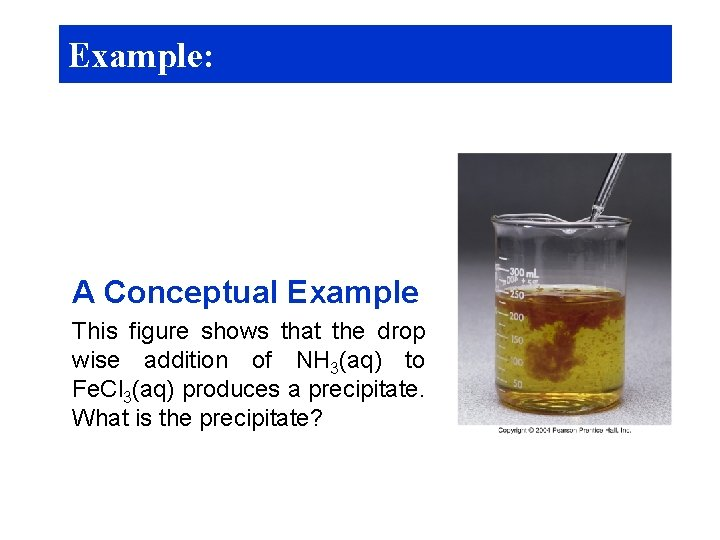Example: A Conceptual Example This figure shows that the drop wise addition of NH 3(aq) to Fe. Cl 3(aq) produces a precipitate. What is the precipitate?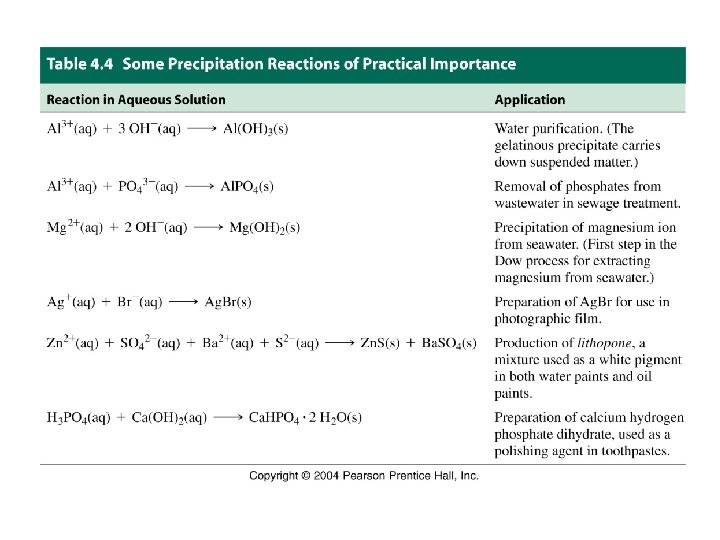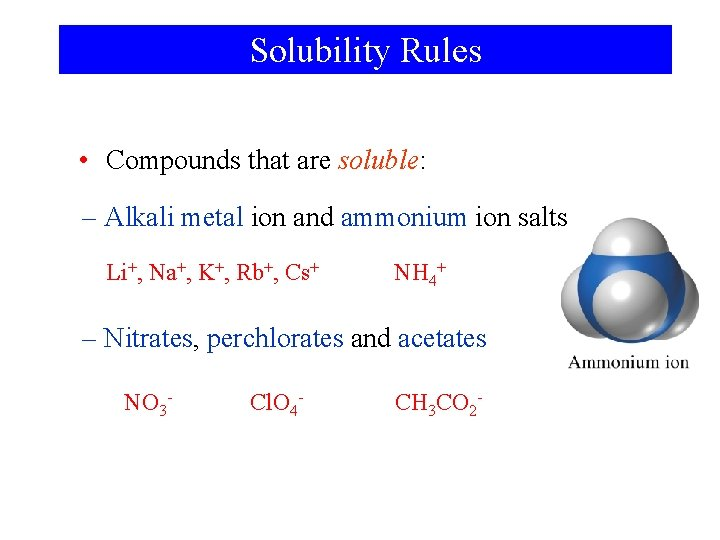Solubility Rules • Compounds that are soluble: – Alkali metal ion and ammonium ion salts Li+, Na+, K+, Rb+, Cs+ NH 4+ – Nitrates, perchlorates and acetates NO 3 - Cl. O 4 - CH 3 CO 2 -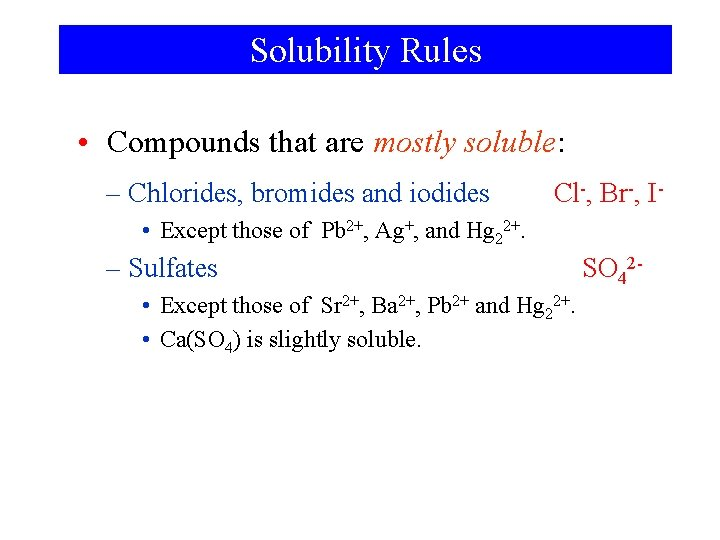Solubility Rules • Compounds that are mostly soluble: – Chlorides, bromides and iodides Cl-, Br-, I- • Except those of Pb 2+, Ag+, and Hg 22+. – Sulfates • Except those of Sr 2+, Ba 2+, Pb 2+ and Hg 22+. • Ca(SO 4) is slightly soluble. SO 42 -Solubility Rules • Compounds that are insoluble: – Hydroxides and sulfides HO-, S 2 - • Except alkali metals, and ammonium salts • Sulfides of alkaline earths are soluble • Hydroxides of Ca 2+, Sr 2+ and Ba 2+ are slightly soluble. – Carbonates and phosphates • Except alkali metal and ammonium salts CO 32 -, PO 43 -Gas Forming Reactions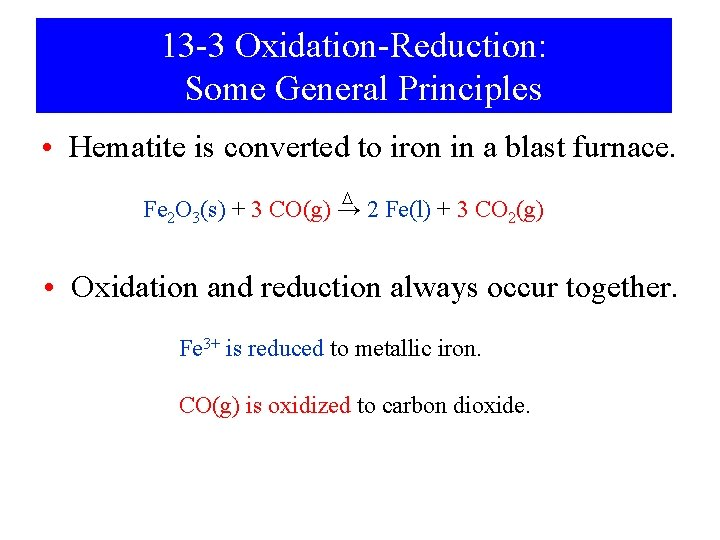13 -3 Oxidation-Reduction: Some General Principles • Hematite is converted to iron in a blast furnace. D Fe 2 O 3(s) + 3 CO(g) → 2 Fe(l) + 3 CO 2(g) • Oxidation and reduction always occur together. Fe 3+ is reduced to metallic iron. CO(g) is oxidized to carbon dioxide.Oxidation State Changes • Assign oxidation states: 3+ 2 - 2+ 2 - D 0 4+ 2 - Fe 2 O 3(s) + 3 CO(g) → 2 Fe(l) + 3 CO 2(g) Fe 3+ is reduced to metallic iron. CO(g) is oxidized to carbon dioxide.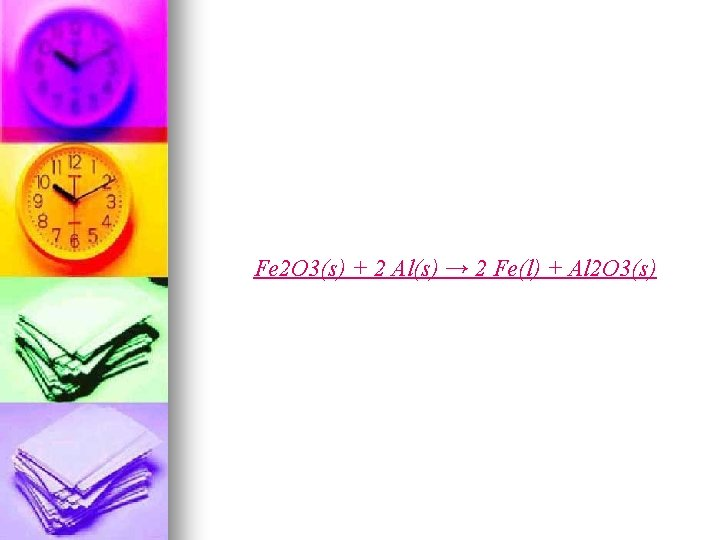Fe 2 O 3(s) + 2 Al(s) → 2 Fe(l) + Al 2 O 3(s)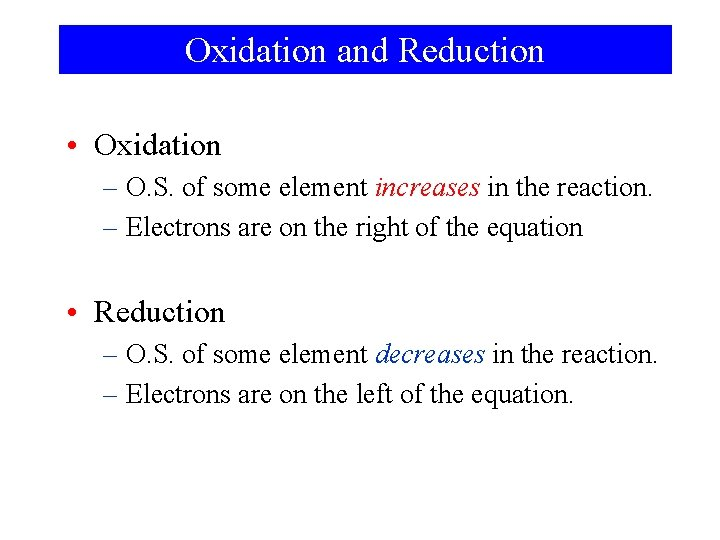Oxidation and Reduction • Oxidation – O. S. of some element increases in the reaction. – Electrons are on the right of the equation • Reduction – O. S. of some element decreases in the reaction. – Electrons are on the left of the equation.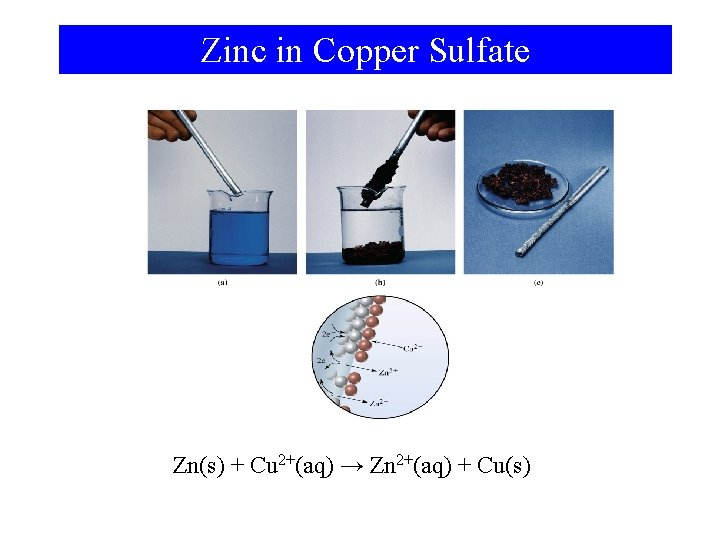Zinc in Copper Sulfate Zn(s) + Cu 2+(aq) → Zn 2+(aq) + Cu(s)Half-Reactions • Represent a reaction by two half-reactions. Oxidation: Zn(s) → Zn 2+(aq) + 2 e- Reduction: Cu 2+(aq) + 2 e- → Cu(s) Overall: Cu 2+(aq) + Zn(s) → Cu(s) + Zn 2+(aq)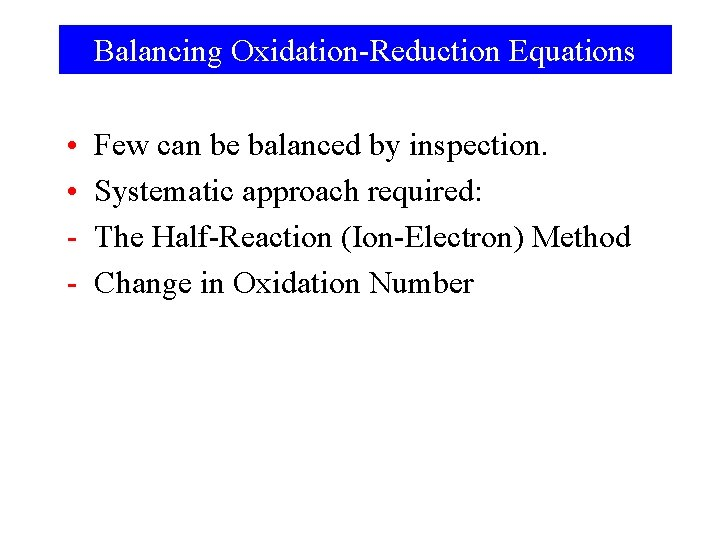Balancing Oxidation-Reduction Equations • • - Few can be balanced by inspection. Systematic approach required: The Half-Reaction (Ion-Electron) Method Change in Oxidation Number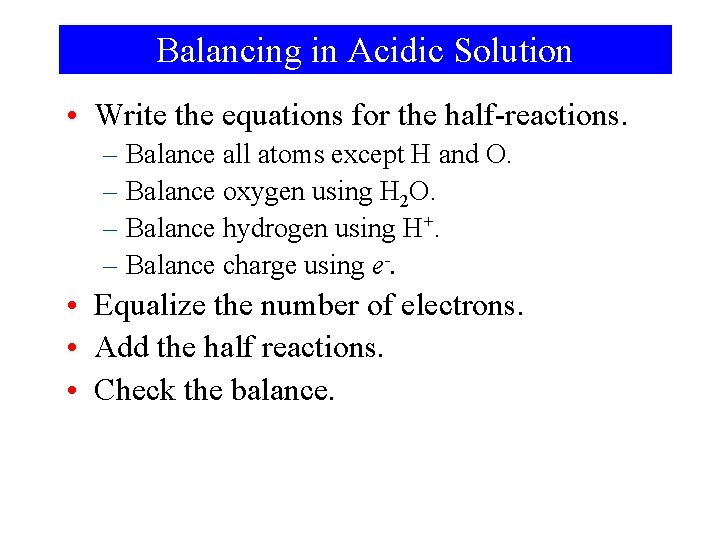Balancing in Acidic Solution • Write the equations for the half-reactions. – Balance all atoms except H and O. – Balance oxygen using H 2 O. – Balance hydrogen using H+. – Balance charge using e-. • Equalize the number of electrons. • Add the half reactions. • Check the balance.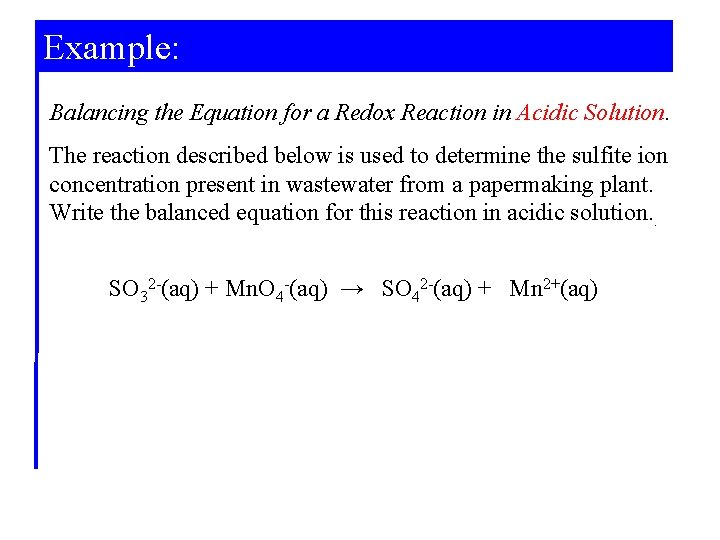Example: Balancing the Equation for a Redox Reaction in Acidic Solution. The reaction described below is used to determine the sulfite ion concentration present in wastewater from a papermaking plant. Write the balanced equation for this reaction in acidic solution. . SO 32 -(aq) + Mn. O 4 -(aq) → SO 42 -(aq) + Mn 2+(aq)Example: Determine the oxidation states: 4+ 6+ 7+ 2+ SO 32 -(aq) + Mn. O 4 -(aq) → SO 42 -(aq) + Mn 2+(aq) Write the half-reactions: SO 32 -(aq) → SO 42 -(aq) + 2 e-(aq) 5 e-(aq) + Mn. O 4 -(aq) → Mn 2+(aq) Balance atoms other than H and O: Already balanced for elements S and Mn.Example: Balance O by adding H 2 O: H 2 O(l) + SO 32 -(aq) → SO 42 -(aq) + 2 e-(aq) 5 e-(aq) + Mn. O 4 -(aq) → Mn 2+(aq) + 4 H 2 O(l) Balance hydrogen by adding H+: H 2 O(l) + SO 32 -(aq) → SO 42 -(aq) + 2 e-(aq) + 2 H+(aq) 8 H+(aq) + 5 e-(aq) +Mn. O 4 -(aq) → Mn 2+(aq) + 4 H 2 O(l) Check that the charges are balanced: Add e- if necessary.Example: Multiply the half-reactions to balance all e-: 5 H 2 O(l) + 5 SO 32 -(aq) → 5 SO 42 -(aq) + 10 e-(aq) + 10 H+(aq) 16 H+(aq) + 10 e-(aq) + 2 Mn. O 4 -(aq) → 2 Mn 2+(aq) + 8 H 2 O(l) Add both equations and simplify: 5 SO 32 -(aq) + 2 Mn. O 4 -(aq) + 6 H+(aq) → 5 SO 42 -(aq) + 2 Mn 2+(aq) + 3 H 2 O(l) Check the balance!Balancing in Basic Solution • OH- appears instead of H+. • Treat the equation as if it were in acid. – Then add OH- to each side to neutralize H+. – Remove H 2 O appearing on both sides of equation. • Check the balance.13 -4 Oxidizing and Reducing Agents. • An oxidizing agent (oxidant): – Contains an element whose oxidation state decreases in a redox reaction • A reducing agent (reductant): – Contains an element whose oxidation state increases in a redox reaction.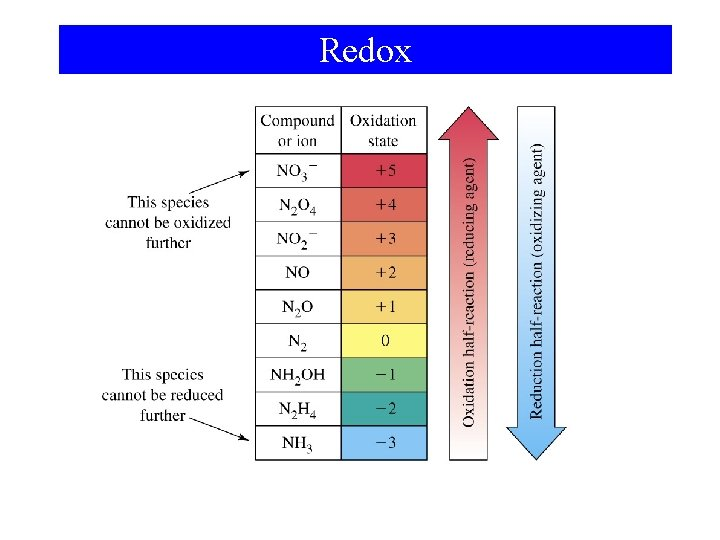Redox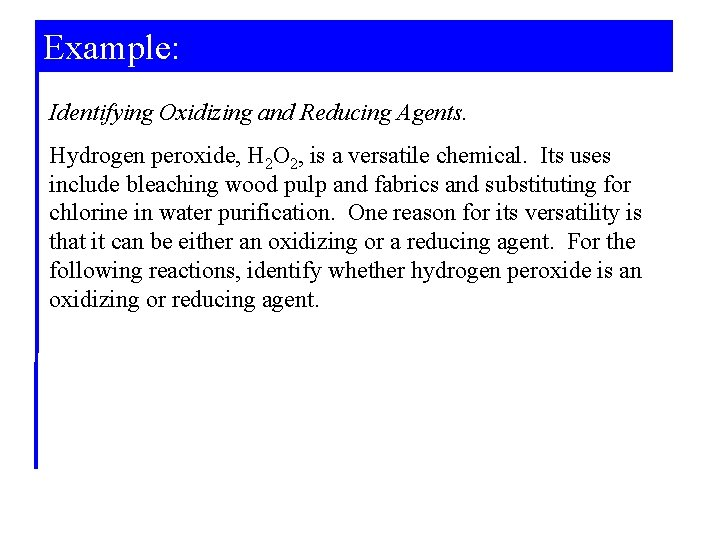Example: Identifying Oxidizing and Reducing Agents. Hydrogen peroxide, H 2 O 2, is a versatile chemical. Its uses include bleaching wood pulp and fabrics and substituting for chlorine in water purification. One reason for its versatility is that it can be either an oxidizing or a reducing agent. For the following reactions, identify whether hydrogen peroxide is an oxidizing or reducing agent.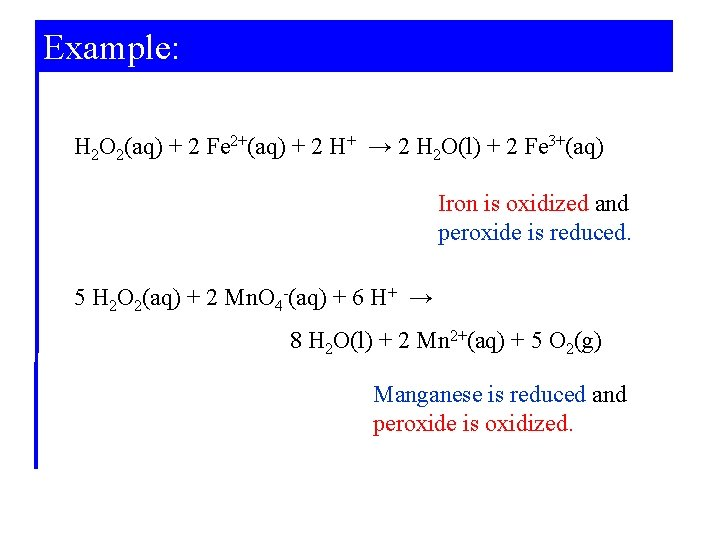Example: H 2 O 2(aq) + 2 Fe 2+(aq) + 2 H+ → 2 H 2 O(l) + 2 Fe 3+(aq) Iron is oxidized and peroxide is reduced. 5 H 2 O 2(aq) + 2 Mn. O 4 -(aq) + 6 H+ → 8 H 2 O(l) + 2 Mn 2+(aq) + 5 O 2(g) Manganese is reduced and peroxide is oxidized.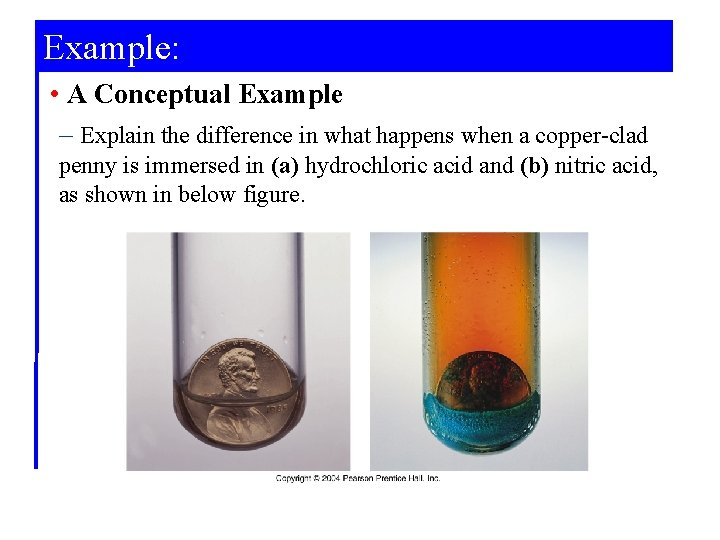Example: • A Conceptual Example – Explain the difference in what happens when a copper-clad penny is immersed in (a) hydrochloric acid and (b) nitric acid, as shown in below figure.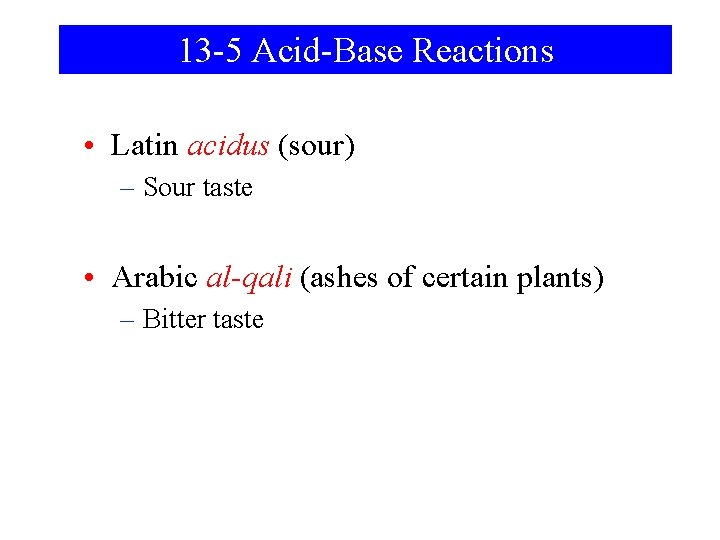13 -5 Acid-Base Reactions • Latin acidus (sour) – Sour taste • Arabic al-qali (ashes of certain plants) – Bitter taste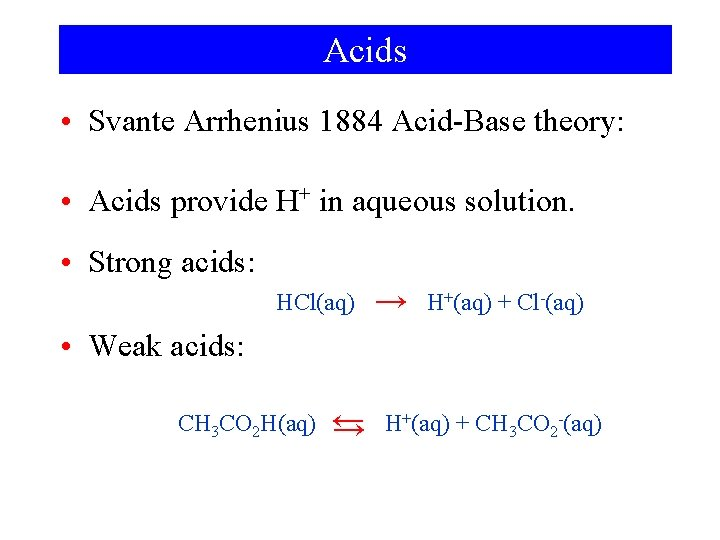Acids • Svante Arrhenius 1884 Acid-Base theory: • Acids provide H+ in aqueous solution. • Strong acids: HCl(aq) → H+(aq) + Cl-(aq) • Weak acids: CH 3 CO 2 H(aq) ← → H+(aq) + CH 3 CO 2 -(aq)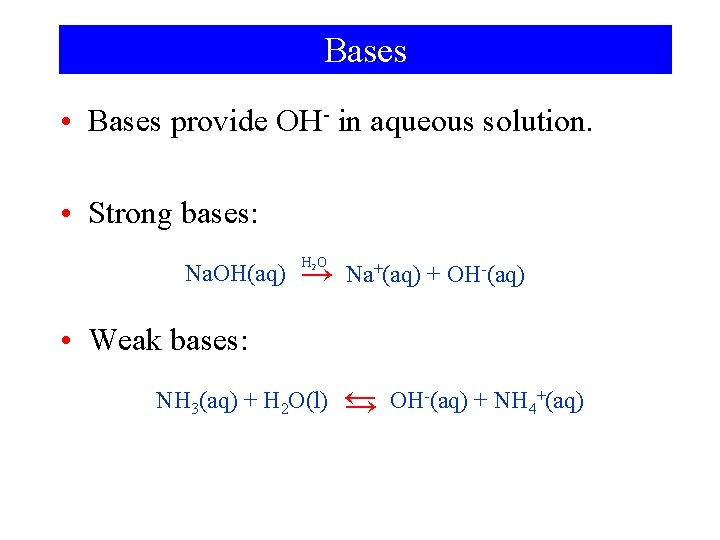Bases • Bases provide OH- in aqueous solution. • Strong bases: Na. OH(aq) H 2 O → Na+(aq) + OH-(aq) • Weak bases: NH 3(aq) + H 2 O(l) ← → OH-(aq) + NH 4+(aq)Recognizing Acids and Bases. • Acids have ionizable hydrogen ions H+. – HCl, CH 3 CO 2 H or HC 2 H 3 O 2 • Bases have OH- combined with a metal ion. KOH or are identified by chemical equations Na 2 CO 3(s) + H 2 O(l)→ HCO 3 -(aq) + 2 Na+(aq) + OH-(aq)More Acid-Base Reactions • Milk of magnesia Mg(OH)2 and acids: Mg(OH)2(s) + 2 H+(aq) → Mg 2+(aq) + 2 H 2 O(l) Mg(OH)2(s) + 2 CH 3 CO 2 H(aq) → Mg 2+(aq) + 2 CH 3 CO 2 -(aq) + 2 H 2 O(l)More Acid-Base Reactions • Limestone and marble and acids: Ca. CO 3(s) + 2 H+(aq) → Ca 2+(aq) + H 2 CO 3(aq) But: H 2 CO 3(aq) → H 2 O(l) + CO 2(g) Ca. CO 3(s) + 2 H+(aq) → Ca 2+(aq) + H 2 O(l) + CO 2(g)Focus On Acid Rain CO 2 + H 2 O H 2 CO 3 + H 2 O HCO 3 - + H 3 O+ 3 NO 2 + H 2 O 2 HNO 3 + NO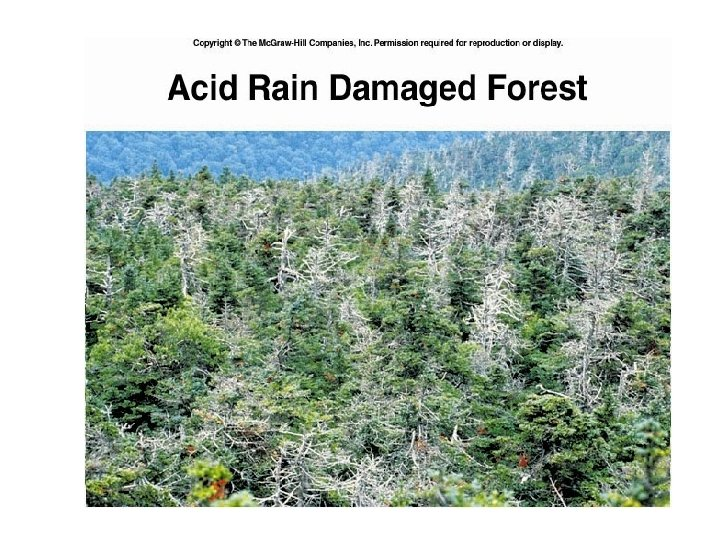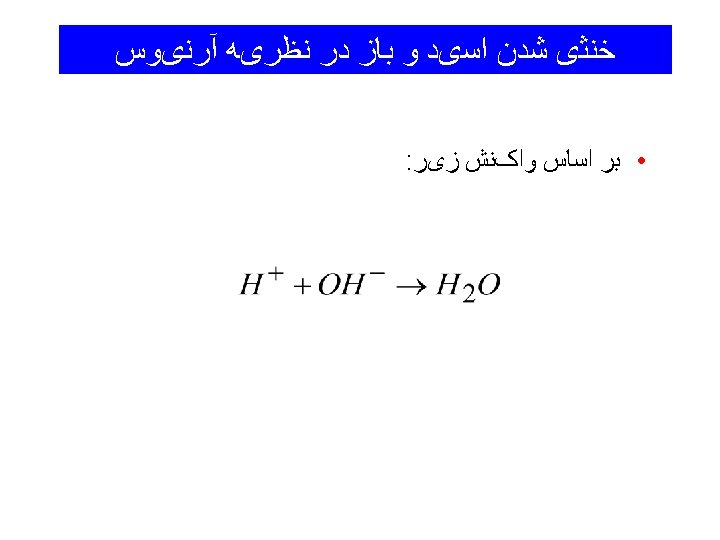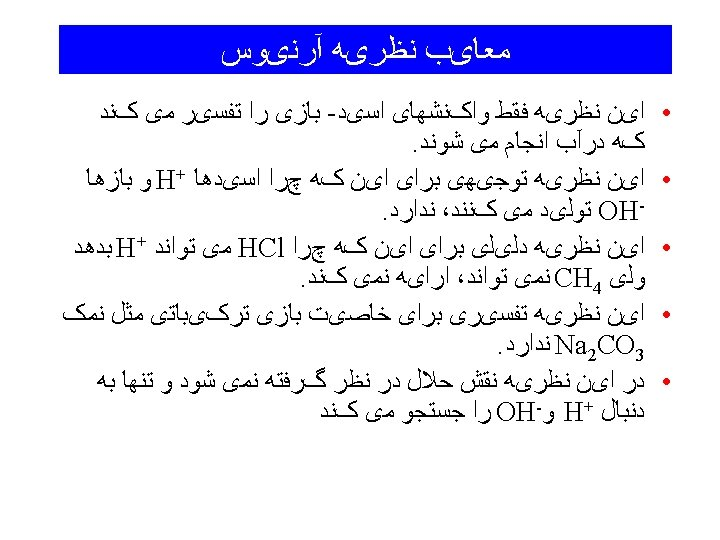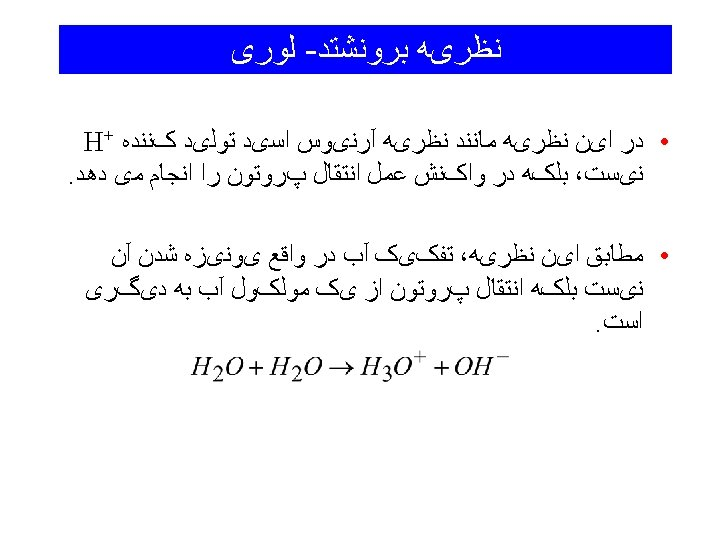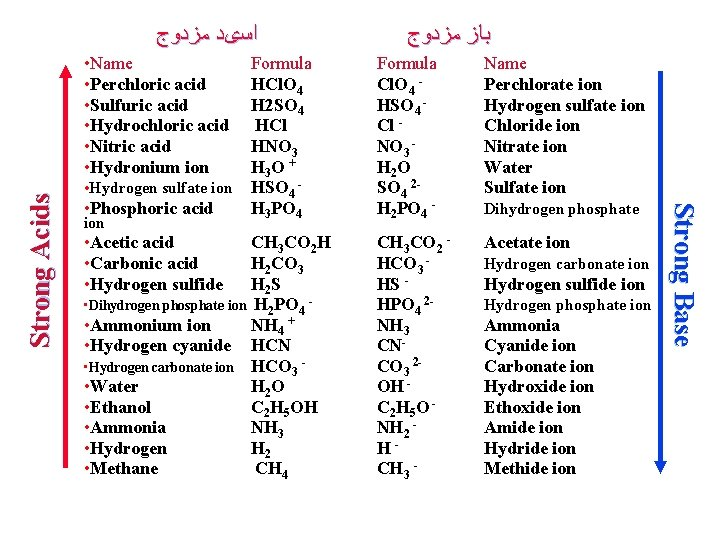ﺍﺳیﺪ ﻣﺰﺩﻭﺝ • Hydrogen sulfate ion • Phosphoric acid ion • Acetic acid • Carbonic acid • Hydrogen sulfide ﺑﺎﺯﻫﺎ ﺍﺳیﺪﻫﺎ ﻭ ﻗﻮﺕ Name Formula HCl. O 4 H 2 SO 4 HCl HNO 3 H 3 O + HSO 4 H 3 PO 4 CH 3 CO 2 H H 2 CO 3 H 2 S • Dihydrogen phosphate ion H 2 PO 4 • Ammonium ion NH 4 + • Hydrogen cyanide HCN • Hydrogen carbonate ion HCO 3 • Water H 2 O • Ethanol C 2 H 5 OH • Ammonia NH 3 • Hydrogen H 2 • Methane CH 4 Cl. O 4 HSO 4 Cl NO 3 H 2 O SO 4 2 H 2 PO 4 - Perchlorate ion Hydrogen sulfate ion Chloride ion Nitrate ion Water Sulfate ion CH 3 CO 2 HCO 3 HS HPO 4 2 NH 3 CNCO 3 2 OH C 2 H 5 O NH 2 HCH 3 - Acetate ion Dihydrogen phosphate Hydrogen carbonate ion Hydrogen sulfide ion Hydrogen phosphate ion Ammonia Cyanide ion Carbonate ion Hydroxide ion Ethoxide ion Amide ion Hydride ion Methide ion Strong Base Strong Acids • Name • Perchloric acid • Sulfuric acid • Hydrochloric acid • Nitric acid • Hydronium ion ﺑﺎﺯ ﻣﺰﺩﻭﺝ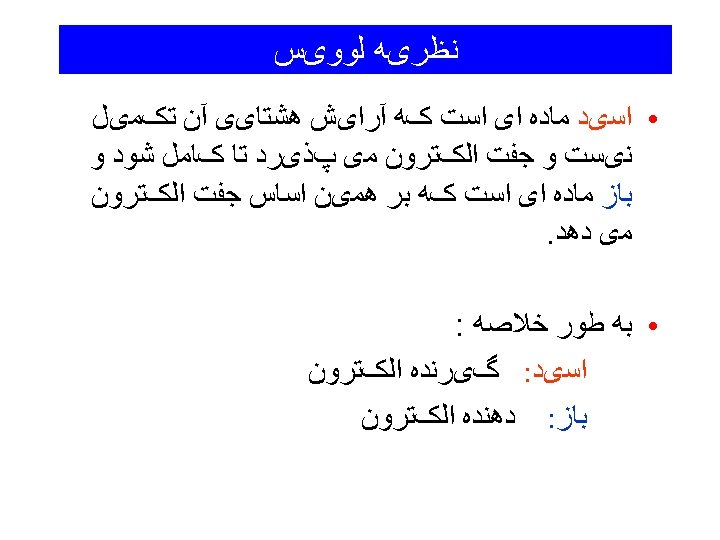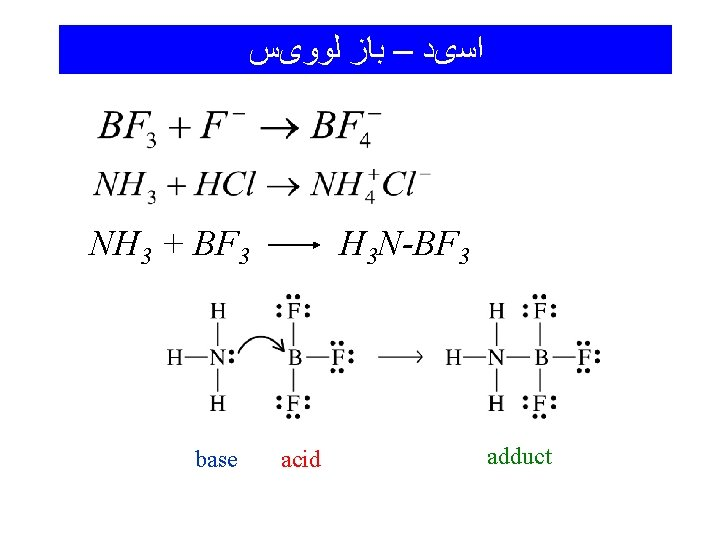ﺍﺳیﺪ – ﺑﺎﺯ ﻟﻮﻭیﺲ NH 3 + BF 3 base H 3 N-BF 3 acid adduct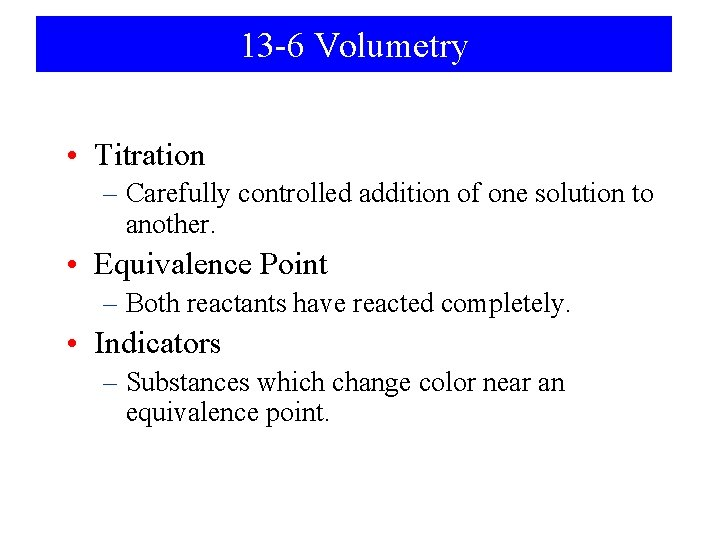13 -6 Volumetry • Titration – Carefully controlled addition of one solution to another. • Equivalence Point – Both reactants have reacted completely. • Indicators – Substances which change color near an equivalence point.Indicators Litmus extract Acid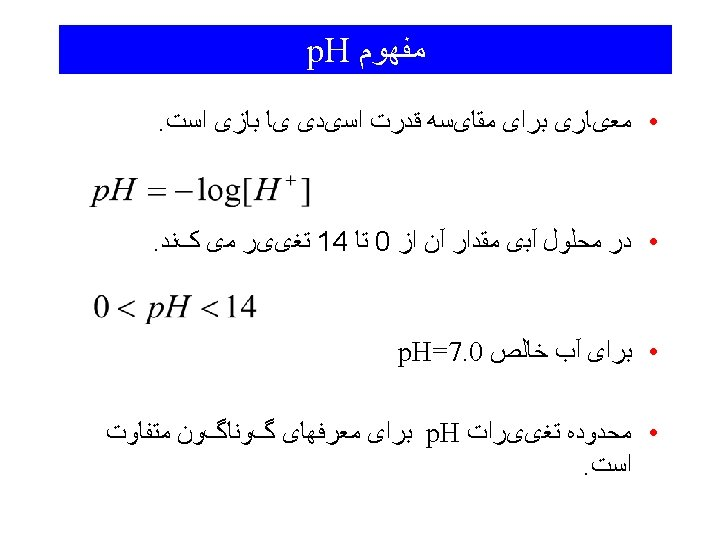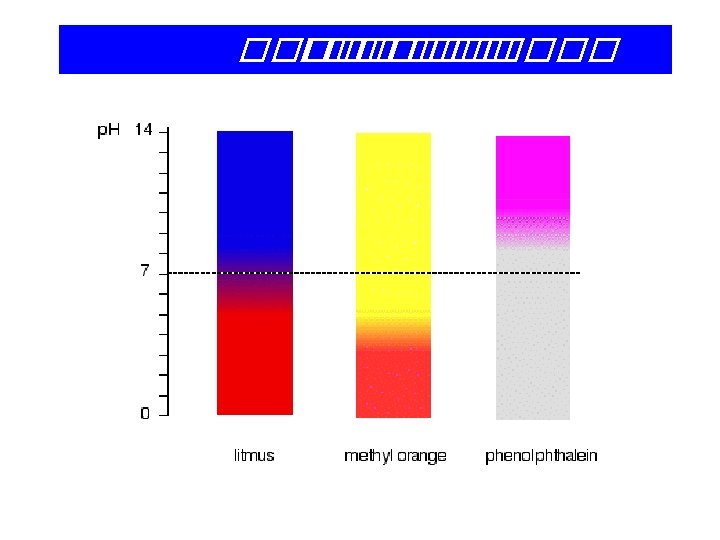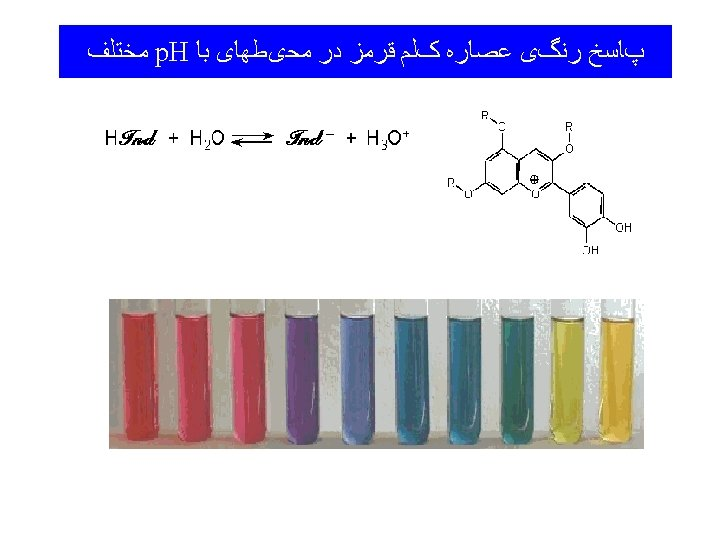Titrations • In a titration, two reactants in solution are combined carefully until they are in stoichiometric proportion. • The objective of a titration is to determine the number of moles, or the number of grams, or the percentage, or the concentration, of the analyte (the sought-for substance in an analysis, the substance we are looking for).Titrations (cont’d) • In a titration, one reactant (the titrant) is placed in a buret. The other reactant is placed in a flask along with a few drops of an indicator. • The titrant is slowly added to the contents of the flask until the indicator changes color (the endpoint). • If the indicator has been chosen properly, the endpoint tells us when the reactants are present in stoichiometric proportion. • A titration may be based on any of the previously discussed types of reactions …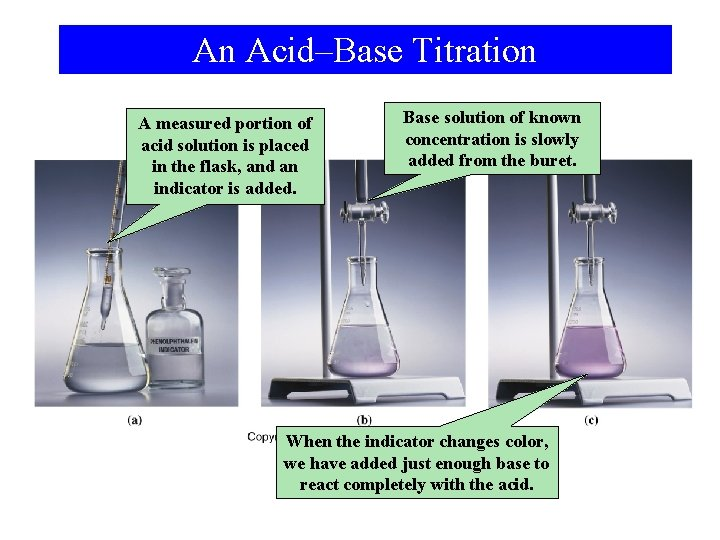An Acid–Base Titration A measured portion of acid solution is placed in the flask, and an indicator is added. Base solution of known concentration is slowly added from the buret. When the indicator changes color, we have added just enough base to react completely with the acid.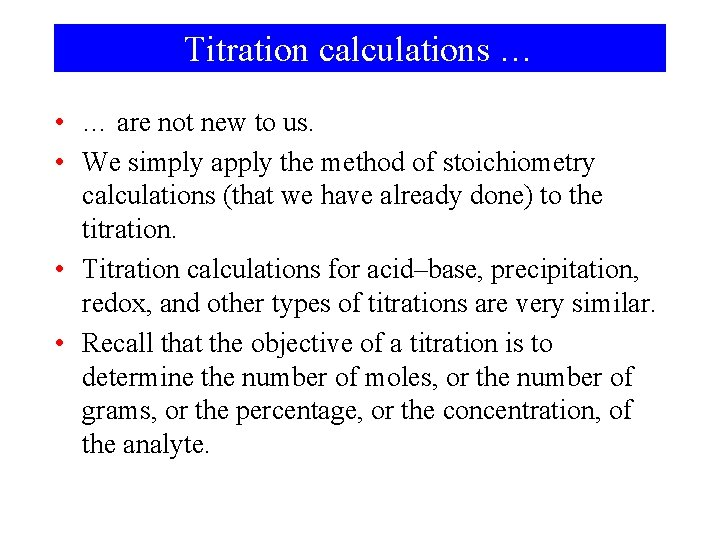Titration calculations … • … are not new to us. • We simply apply the method of stoichiometry calculations (that we have already done) to the titration. • Titration calculations for acid–base, precipitation, redox, and other types of titrations are very similar. • Recall that the objective of a titration is to determine the number of moles, or the number of grams, or the percentage, or the concentration, of the analyte.Example: What volume (m. L) of 0. 2010 M Na. OH is required to neutralize 20. 00 m. L of 0. 1030 M HCl in an acid–base titration? Example: A 10. 00 -m. L sample of an aqueous solution of calcium hydroxide is neutralized by 23. 30 m. L of 0. 02000 M HNO 3(aq). What is the molarity of the calcium hydroxide solution?A Precipitation Titration … with silver nitrate solution. An unknown concentration of chloride ion is being titrated … … the next drop of Ag+ solution produces brick-red silver dichromate. The indicator is orange dichromate ion; white Ag. Cl precipitates. When the chloride has reacted completely …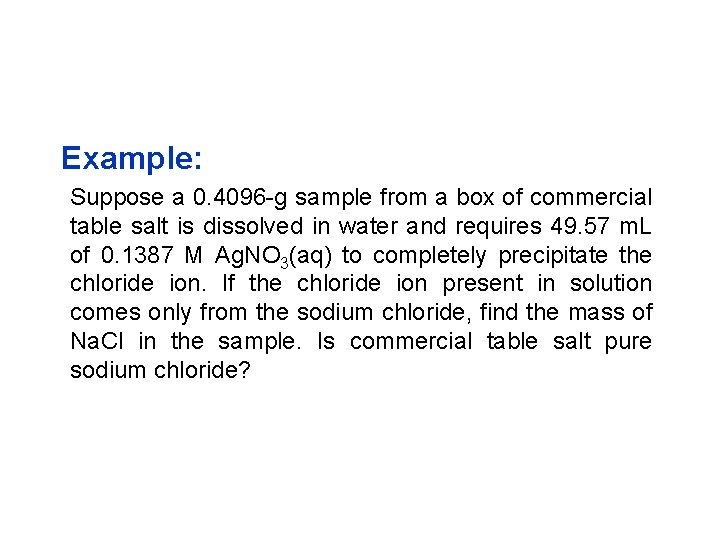Example: Suppose a 0. 4096 -g sample from a box of commercial table salt is dissolved in water and requires 49. 57 m. L of 0. 1387 M Ag. NO 3(aq) to completely precipitate the chloride ion. If the chloride ion present in solution comes only from the sodium chloride, find the mass of Na. Cl in the sample. Is commercial table salt pure sodium chloride?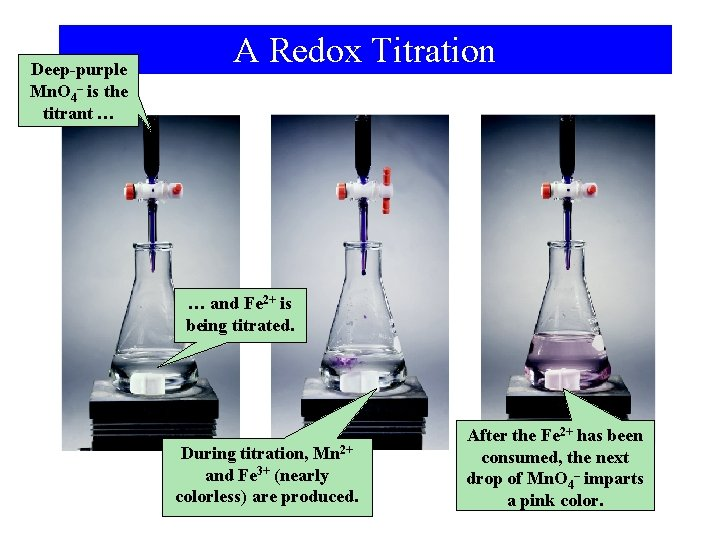Deep-purple Mn. O 4– is the titrant … A Redox Titration … and Fe 2+ is being titrated. During titration, Mn 2+ and Fe 3+ (nearly colorless) are produced. After the Fe 2+ has been consumed, the next drop of Mn. O 4– imparts a pink color.Example: Standardizing a Solution for Use in Redox Titrations. A piece of iron wire weighing 0. 1568 g is converted to Fe 2+(aq) and requires 26. 42 m. L of a KMn. O 4(aq) solution for its titration. What is the molarity of the KMn. O 4(aq)? 5 Fe 2+(aq) + Mn. O 4 -(aq) + 8 H+(aq) → 4 H 2 O(l) + 5 Fe 3+(aq) + Mn 2+(aq)Example: 5 Fe 2+(aq) + Mn. O 4 -(aq) + 8 H+(aq) → 4 H 2 O(l) + 5 Fe 3+(aq) + Mn 2+(aq) Determine KMn. O 4 consumed in the reaction: n. KMn. O 4 Determine the concentration: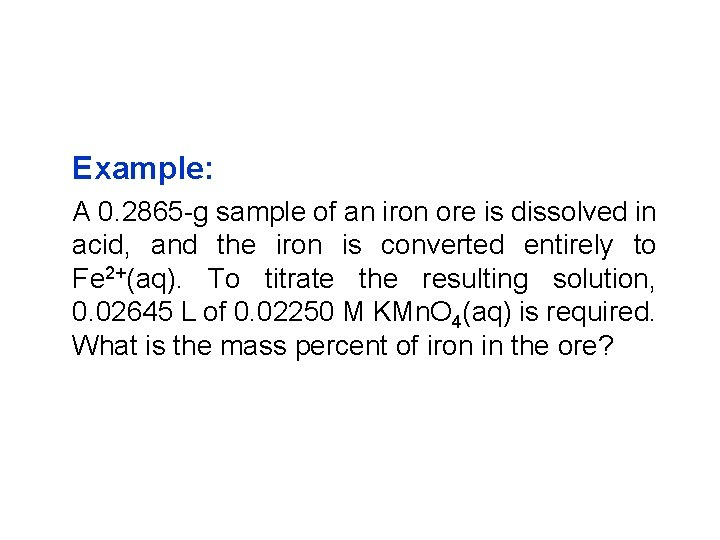Example: A 0. 2865 -g sample of an iron ore is dissolved in acid, and the iron is converted entirely to Fe 2+(aq). To titrate the resulting solution, 0. 02645 L of 0. 02250 M KMn. O 4(aq) is required. What is the mass percent of iron in the ore?Cumulative Example Sodium nitrite is used in the production of fabric dyes, as a meat preservative, as a bleach for fibers, and in photography. It is prepared by passing nitrogen monoxide gas and oxygen gas into an aqueous solution of sodium carbonate. Carbon dioxide gas is the other product of the reaction. (a) Write a balanced equation for the reaction. (b) What mass of sodium nitrite should be produced in the reaction of 748 g of Na 2 CO 3, with the other reactants in excess? (c) In another preparation, the reactants are 225 m. L of 1. 50 M Na 2 CO 3(aq), 22. 1 g nitrogen monoxide, and excess O 2. What mass of sodium nitrite should be produced if the reaction has a yield of 95. 1%?Chapter 13 Questions 3, 6, 12, 15, 17, 20, 21, 24, 36, 40, 44, 50, 58, 59, 62, 68Example: Predict whether a precipitation reaction will occur in each of the following cases. If so, write a net ionic equation for the reaction. (a) Na 2 SO 4(aq) + Mg. Cl 2(aq) ? (b) (NH 4)2 S(aq) + Cu(NO 3)2(aq) ? (c) K 2 CO 3(aq) + Zn. Cl 2(aq) ?Example: A Conceptual Example This figure shows that the drop wise addition of NH 3(aq) to Fe. Cl 3(aq) produces a precipitate. What is the precipitate?